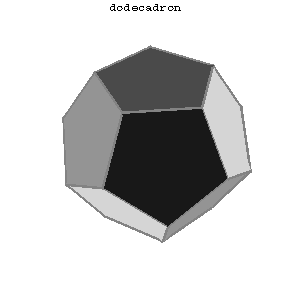# Dodecahedron

One of the five types of regular polyhedra. A dodecahedron has 12 (pentagonal) faces, 30 edges and 20 vertices (with three edges meeting at each vertex). If $a$ is the edge length of a dodecahedron, its volume is
$$v=\frac{a^3}{4}(15+7\sqrt5)\approx7.6631a^3.$$